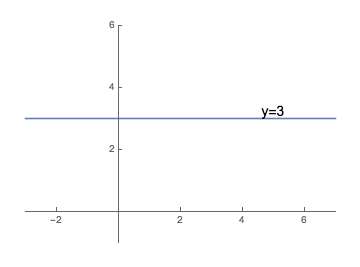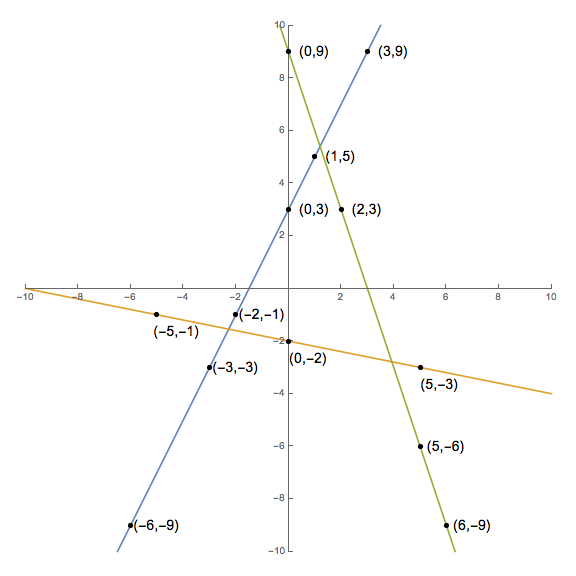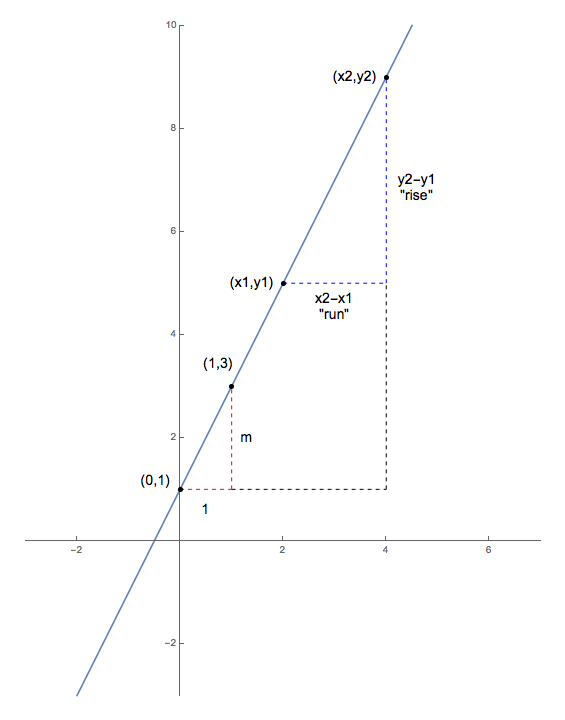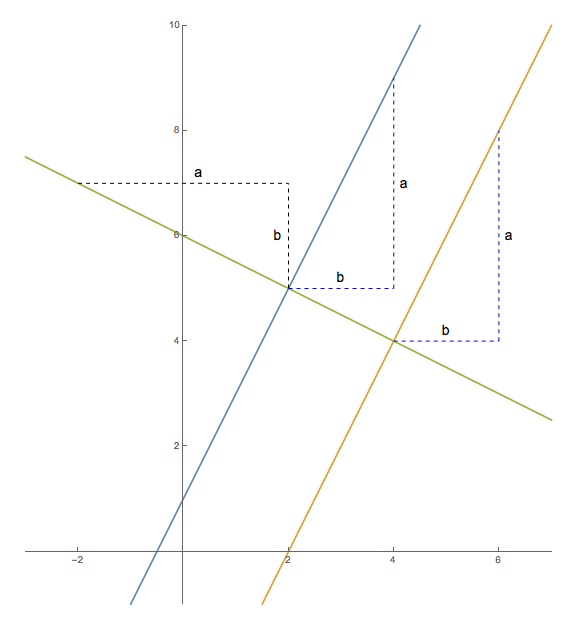## Lines

Polynomial functions of degree zero (i.e., functions that take the form $f(x)=c$ for some constant $c$) are not terribly interesting. Since the output for any input $x$ is $c$, the graph consists of all points at height $c$ in the coordinate plane. Consider $f(x)=3$, whose graph is shown below. As can be seen, the graph is just a horizontal line with $y$-intercept $(0,3)$. All constant functions graph as horizontal lines in a similar manner.Let us turn, then, our attention to polynomial functions of degree one...

As one can see in the picture below, if we plot a few points on the graph of a function of the form $f(x) = mx + b$ for some constants $m$ and $b$, something very interesting happens -- all of the points end up falling on the same line! Because of this, we call functions of this form linear functions.

--- $g(x)=2x+3$    --- $h(x)=-\frac{1}{5}x-2$    --- $q(x)=-3x+9$To get a feel for why this happens, consider one point on the graph $y=f(x)$ that is really easy to calculate -- the $y$-intercept. Noting that $f(0)=b$ tells us this intercept is $(0,b)$. Beginning at that location notice how each time we increase $x$ by $1$, the height of the corresponding point on the graph of $y=f(x)$ moves up $m$ units (or down, if $m$ is negative).

Algebraically, we are just observing that $$\begin{array}{rcl} f(x+1) & = & m(x+1) + b\\ & = & mx + m + b\\ & = & mx + b + m\\ & = & f(x) + m \end{array}$$ Of course, such a steady ascent (or descent) can't help but be a linear one.

(As a nice mnemonic, note the choice of letters to represent the constants of our function above -- we begin at the $y$-intercept $(0,b)$ and move $m$ units upward for every unit move to the right.)

Note that larger values of $m$ result in a steeper slope from left to right since we move upward more per unit increase in our $x$-coordinate. As such, we call the value of $m$ the slope of the line.

Positive slopes are then clearly associated with lines that rise in height from left to right (since with each move to the right, we are adding a positive value to the $y$-coordinate), while negative slopes are associated with lines that fall in height from left to right.

If the slope is zero, then the points of the associated line fails to move upward or downward as the $x$-coordinate changes. Equivalently, if $m=0$, the line must be horizontal.

Given any two distinct points on a given line, we can find the related slope very quickly by using similar triangles, as we can see below.Supposing the two points in question have coordinates $(x_1,y_1)$ and $(x_2,y_2)$, we add some vertical and horizontal dashed lines to form three right triangles -- one large triangle, one with red legs, and one with blue legs. Quick inspection of some of the angles formed tells us that any two of these triangles are similar. Given that the red and blue legged right triangles are similar, and as a consequence the ratios of corresponding pairs of side lengths must agree, note that we have

$$m = \frac{m}{1} = \frac{y_2-y_1}{x_2-x_1}$$ or, perhaps more memorably, $$\textrm{slope } m = \frac{\textrm{rise}}{\textrm{run}}$$

### Parallel and Perpendicular Lines

Something that will be of interest to us later in calculus is the relationship between the slopes of parallel and perpendicular lines. As the graphic below clearly shows, the slopes of parallel lines agree in value (note the blue and orange slopes are both $m=\frac{a}{b}$). One can use similar triangles to prove this, and the reader is challenged to construct this proof. (Don't worry -- it's a fairly easy argument...)The slopes of perpendicular lines, however, are more interesting. We can think of drawing a perpendicular to a given line through a given point, as rotating the given line $90^{\circ}$ about that point. Consider rotating the blue line $90^{\circ}$ counter-clockwise about the point $(2,5)$ above. See how it lands on the green line? Thought of in this way, if the given line had a "rise" and "run" of $a$ and $b$ , respectively (as our blue line does) -- then the perpendicular line must have a "rise" and "run" of $-b$ and $a$, respectively (which our green line does).

As such, the slopes of a line and line perpendicular to it, $m = \frac{a}{b}$ and $m_{\perp} = -\frac{b}{a}$, are always negative reciprocals of one another.

### Forms for the Equation of a Line

Certainly, the graph of $y=mx+b$ is a line, given the above discussion. Since the slope $m$ and $y$-intercept $(0,b)$ are immediately obvious when the equation is written in this way, we call this the slope-intercept form of the line.

However, there are other equations reducible to the slope-intercept form that can sometimes be even more helpful in certain situations.

For example, suppose that we need the equation for a line that we know passes through some point $(x_0,y_0)$ and has some slope $m$. (By the way, this is actually a very common occurrence in Calculus.)

We already have the slope $m$, so we can find the slope-intercept form of this line by solving for $b$, the height of the $y$-intercept, in the following equation:

$$y_0 = m x_0 + b$$

Clearly, $$b = y_0 - m x_0$$

So, the slope-intercept form of the line must be given by $$y = mx + (y_0 - mx_0)$$

Subtracting $y_0$ from both sides and factoring out an $m$ gives us an equation equivalent to the slope-intercept form:

$$y-y_0 = m(x-x_0)$$

The above then provides an immediate equation for the line that goes through a given point $(x_0,y_0)$ with a given slope $m$. As an example, if a line goes through $(3,-2)$ with slope $5$, then an equation for this line is given by $(y+2) = 5(x-3)$. Because we can produce such an equation immediately from knowledge of only a single point on the line and the slope of the line, we say that such equations are in point-slope form.

Another form that proves useful is the general form: $$Ax + By = C$$ where $A$, $B$, and $C$ are some constants. There are a couple of advantages to this form...

First, this form can describe vertical lines (as an example, $1\cdot x + 0 \cdot y = 2$, or more simply $x=2$), whereas the previous two forms are unable to do this. (Recall the slope-intercept form was based on a function, and vertical lines are not functions.)

Second, any line that can be written in slope-intercept form with a rational $m$ and $b$ can be written in general form with integer values for $A$, $B$, and $C$ -- which can make working with such equations easier in some sense.

As an example, suppose we wish to convert the linear equation $y = \frac{-2}{3}x + 7$ into general form.

Simply multiply both sides by $3$ to clear the fractions, and then move the $x$-term to the other side by subtracting it from both sides to arrive at:

$$2x + 3y = 21$$

Additionally, finding both the $x$ and $y$-intercepts is easy if the equation is in general form -- simply let $x$ or $y$ be zero and solve for the other coordinate.

As an example, suppose we have the line given by $3x - 4y = 12$. We can easily see that if $x=0$, then $y=-3$, and if $y=0$, then $x=4$. This tells us we have an $x$-intercept of $(4,0)$ and a $y$-intercept of $(0,-3)$.

Finding the slope of a line in general form is fairly simple as well. Note that if $$Ax + By = C$$ then we can quickly solve for $y$, putting the equation back into slope-intercept form, $$y=\frac{-A}{B}x + \frac{C}{B}$$ which reveals that the slope of the line is always given by $$m=\frac{-A}{B}$$